# 3D Reflection in Computer Graphics | Definition | Examples

## 3D Transformations in Computer Graphics-

We have discussed-

• Transformation is a process of modifying and re-positioning the existing graphics.
• 3D Transformations take place in a three dimensional plane.

In computer graphics, various transformation techniques are-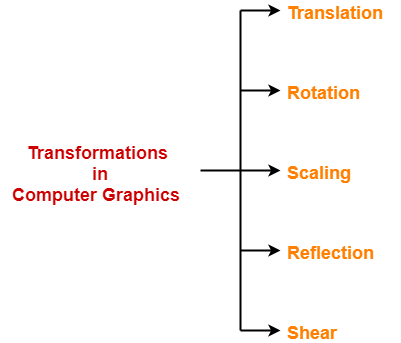In this article, we will discuss about 3D Reflection in Computer Graphics.

## 3D Reflection in Computer Graphics-

• Reflection is a kind of rotation where the angle of rotation is 180 degree.
• The reflected object is always formed on the other side of mirror.
• The size of reflected object is same as the size of original object.

Consider a point object O has to be reflected in a 3D plane.

Let-

• Initial coordinates of the object O = (Xold, Yold, Zold)
• New coordinates of the reflected object O after reflection = (Xnew, Ynew,Znew)

In 3 dimensions, there are 3 possible types of reflection-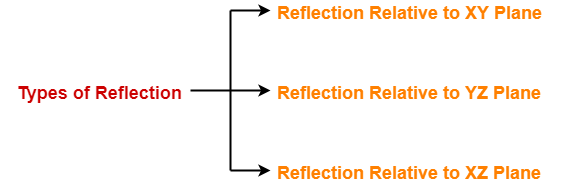• Reflection relative to XY plane
• Reflection relative to YZ plane
• Reflection relative to XZ plane

### Reflection Relative to XY Plane:

This reflection is achieved by using the following reflection equations-

• Xnew = Xold
• Ynew = Yold
• Znew = -Zold

In Matrix form, the above reflection equations may be represented as-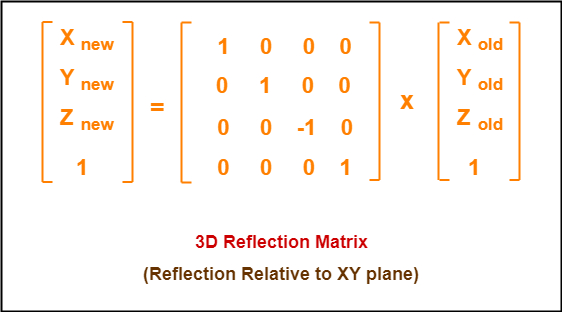### Reflection Relative to YZ Plane:

This reflection is achieved by using the following reflection equations-

• Xnew = -Xold
• Ynew = Yold
• Znew = Zold

In Matrix form, the above reflection equations may be represented as-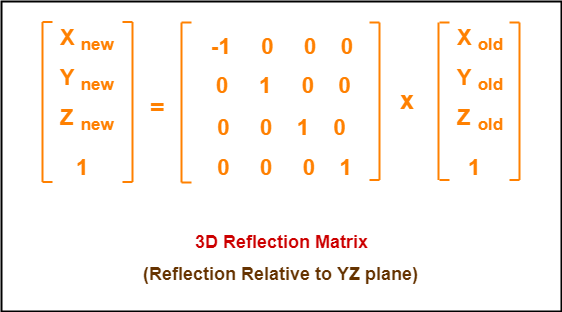### Reflection Relative to XZ Plane:

This reflection is achieved by using the following reflection equations-

• Xnew = Xold
• Ynew = -Yold
• Znew = Zold

In Matrix form, the above reflection equations may be represented as-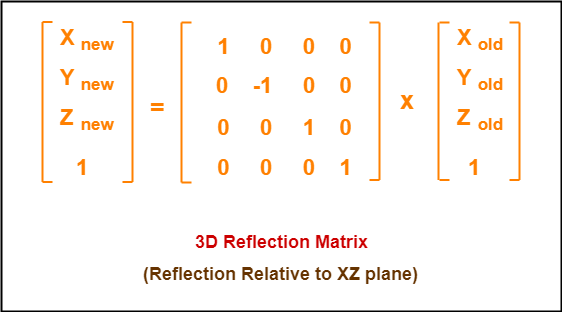## Problem-01:

Given a 3D triangle with coordinate points A(3, 4, 1), B(6, 4, 2), C(5, 6, 3). Apply the reflection on the XY plane and find out the new coordinates of the object.

## Solution-

Given-

• Old corner coordinates of the triangle = A (3, 4, 1), B(6, 4, 2), C(5, 6, 3)
• Reflection has to be taken on the XY plane

### For Coordinates A(3, 4, 1)

Let the new coordinates of corner A after reflection = (Xnew, Ynew, Znew).

Applying the reflection equations, we have-

• Xnew = Xold = 3
• Ynew = Yold = 4
• Znew = -Zold = -1

Thus, New coordinates of corner A after reflection = (3, 4, -1).

### For Coordinates B(6, 4, 2)

Let the new coordinates of corner B after reflection = (Xnew, Ynew, Znew).

Applying the reflection equations, we have-

• Xnew = Xold = 6
• Ynew = Yold = 4
• Znew = -Zold = -2

Thus, New coordinates of corner B after reflection = (6, 4, -2).

### For Coordinates C(5, 6, 3)

Let the new coordinates of corner C after reflection = (Xnew, Ynew, Znew).

Applying the reflection equations, we have-

• Xnew = Xold = 5
• Ynew = Yold = 6
• Znew = -Zold = -3

Thus, New coordinates of corner C after reflection = (5, 6, -3).

Thus, New coordinates of the triangle after reflection = A (3, 4, -1), B(6, 4, -2), C(5, 6, -3).

## Problem-02:

Given a 3D triangle with coordinate points A(3, 4, 1), B(6, 4, 2), C(5, 6, 3). Apply the reflection on the XZ plane and find out the new coordinates of the object.

## Solution-

Given-

• Old corner coordinates of the triangle = A (3, 4, 1), B(6, 4, 2), C(5, 6, 3)
• Reflection has to be taken on the XZ plane

### For Coordinates A(3, 4, 1)

Let the new coordinates of corner A after reflection = (Xnew, Ynew, Znew).

Applying the reflection equations, we have-

• Xnew = Xold = 3
• Ynew = -Yold = -4
• Znew = Zold = 1

Thus, New coordinates of corner A after reflection = (3, -4, 1).

### For Coordinates B(6, 4, 2)

Let the new coordinates of corner B after reflection = (Xnew, Ynew, Znew).

Applying the reflection equations, we have-

• Xnew = Xold = 6
• Ynew = -Yold = -4
• Znew = Zold = 2

Thus, New coordinates of corner B after reflection = (6, -4, 2).

### For Coordinates C(5, 6, 3)

Let the new coordinates of corner C after reflection = (Xnew, Ynew, Znew).

Applying the reflection equations, we have-

• Xnew = Xold = 5
• Ynew = -Yold = -6
• Znew = Zold = 3

Thus, New coordinates of corner C after reflection = (5, -6, 3).

Thus, New coordinates of the triangle after reflection = A (3, -4, 1), B(6, -4, 2), C(5, -6, 3).

To gain better understanding about 3D Reflection in Computer Graphics,

Watch this Video Lecture

Next Article- 3D Shearing in Computer Graphics

Get more notes and other study material of Computer Graphics.

Watch video lectures by visiting our YouTube channel LearnVidFun.

SummaryArticle Name
3D Reflection in Computer Graphics | Definition | Examples
Description
Reflection in Computer Graphics is a kind of rotation where the angle of rotation is 180 degree. 3D Reflection takes place in 3D plane. Reflection in Computer Graphics Definition, Solved Examples and Problems.
Author
Publisher Name
Gate Vidyalay
Publisher Logo